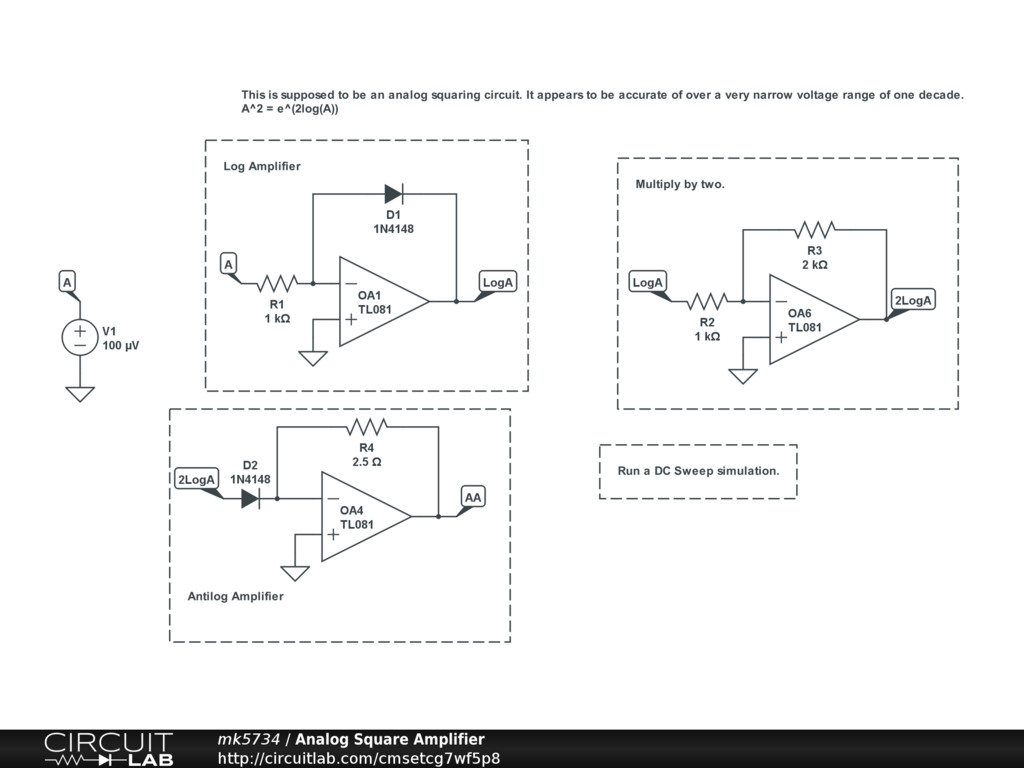## Analog Voltage Squarer

 Hello everyone! I'm trying to simulate a circuit (that I designed, for better or worse) that squares the input voltage. I run the input voltage through a log amplifier, then through an amplifier which multiplies it by two, and then through an antilog amplifier which should result in the square of the input voltage.If I set R3 to 1K, then the multiplication is by one, which results in the output equaling the input voltage, which is correct. However, if I set R3 to 2K, then I get some very crazy voltage outputs. Also, if I set R3 to 500 ohms, this should result in the square root of the input, but doesn't appear to. What am I doing wrong? Could it be the voltage range that it works over? Thanks! by mk5734 September 14, 2018 I think I figured it out. It only appears to work over a very narrow range of input voltages. by mk5734 September 14, 2018